# Lecture - Visualising Numbers (Part 2)

## Sums of Squares

We have seen last time, that the sum $\sum_{i=1}^n i$ counts the number of lattice points in triangles with slope 1. What geometric object is associated with $\sum_{i=1}^n i^2?$

$\sum_{i=1}^n i^2$ counts the number of lattice points in pyramids.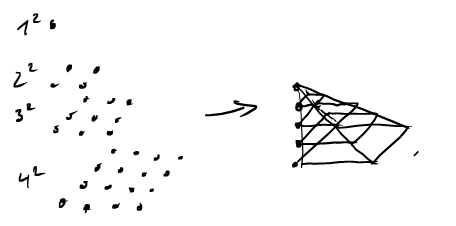Using this insight it is possible to derive the formula $\sum_{i=1}^n i^2=\frac{n(n+2)(2n+1)}{6}.$

We will not do that right now, but instead consider pyramids "with rational slope".

## Pyramids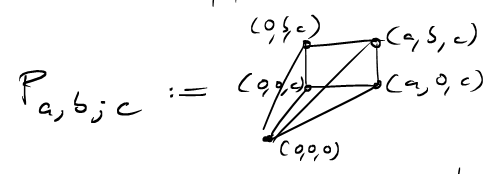Notice that by reflection $P_{a,b;c}=P_{b,a;c}$, which means that these pyramids are symmetric in the first two arguments but not in the last. Now, how many lattice points are in $P_{a,b;c}?$ To this end, let us intersect the pyramid with a hyperplane, where the last coordinate is fixed.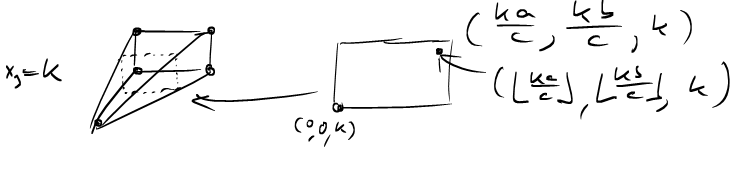The top-right lattice point in each slice has coordinates $(\lfloor \frac{ka}{c} \rfloor, \lfloor \frac{kb}{c} \rfloor, k)$ and so the pyramid contains $L(P_{a,b;c})= \sum_{k=0}^c (\left\lfloor \frac{ka}{c} \right\rfloor + 1) (\left\lfloor \frac{kb}{c} \right\rfloor + 1)$ lattice points.

In this sum, the "$+1$s" are kind of annoying, so can we modify the pyramids so that the lattice point count is exactly $\sum_{k=0}^{c-1} \left\lfloor \frac{ka}{c} \right\rfloor \cdot \left\lfloor \frac{kb}{c} \right\rfloor$?

One solution is to remove the hyperplanes given by $x_1=0$, $x_2=0$ and $x-3=c$ from the pyramid. If $\hat{P}_{a,b;c}$ is the "half-open" pyramid obtained by removing these hyperplanes from $P_{a,b;c}$, then we have effectively removed one column and one row of lattice points from each "slice" and so, indeed, $L(\hat{P}_{a,b;c}) = \sum_{k=0}^{c-1} \left\lfloor \frac{ka}{c} \right\rfloor \cdot \left\lfloor \frac{kb}{c} \right\rfloor.$

Now, let’s try to do the same trick we did last time:

Can we build a simple geometric shape out of pyramids of this type in order to obtain a formula for these sums?

When last time, we built a rectangle out of triangles, this time, we are going to build a right-rectangular prism out of pyramids! (By the way, right-rectangular prims are called quader in German, which is a much shorter word, so I am going to stick to that one.) The idea is this: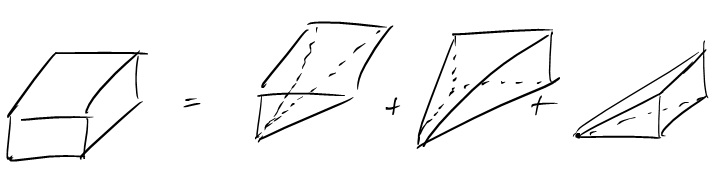Let us consider the easy case first, where $a,b,c$ are pairwise relatively prime, that is, $\text{gcd}(a,b)=\text{gcd}(b,c)=\text{gcd}(a,c)=1.$

How many lattice points are now on the triangles where two of the pyramids intersect?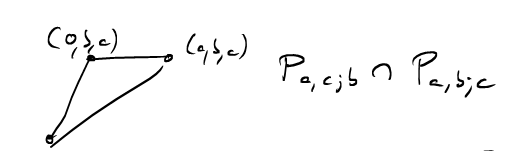In the relatively prime case, the only lattice points on this triangle are the origin and those on the line from $(0,b,c)$ to $(a,b,c)$. But all of these lie on hyperplanes that have been removed from our half-open pyramids! So the intersection of any two half-open pyramids does not contain any lattice points!

This means that in the relatively prime case, we will not have any double counting. Putting the three half-open pyramids together, we obtain the open quader $\hat{P}_{a,b;c} \cup \hat{P}_{a,c;b} \cup \hat{P}_{b,c;a} = (0,a) \times (0,b) \times (0,c)$ where the union is disjoint, and so we get the formula $L(\hat{P}_{a,b;c}) + L(\hat{P}_{a,c;b}) + L(\hat{P}_{b,c;a}) = L((0,a) \times (0,b) \times (0,c))$ which yields $\sum_{k=0}^{c-1} \left\lfloor \frac{ka}{c} \right\rfloor \cdot \left\lfloor \frac{kb}{c} \right\rfloor + \sum_{k=0}^{b-1} \left\lfloor \frac{ka}{b} \right\rfloor \cdot \left\lfloor \frac{kc}{b} \right\rfloor + \sum_{k=0}^{a-1} \left\lfloor \frac{kb}{a} \right\rfloor \cdot \left\lfloor \frac{kc}{a} \right\rfloor = (a-1)(b-1)(c-1).$

This is a typical example of a reciprocity theorem. This term stems from the fact that fractions in which numerator and denominator have been exchanged are related, but I would like to use the term for a much wider class of theorems:

A reciprocity theorem is a theorem that puts several complicated functions (that, individually, have no simple form) in a simple relation.

We have been dealing with number theoretic functions here, but reciprocity theorems also exist for combinatorial counting functions, as we will see in a couple of weeks. In both cases, the message is that geometry helps finding reciprocity theorems.

## Dedekind sums

Before we deal with the case where $a,b,c$ are not relatively prime, let us first take a closer look at the sums $\sum_{k=0}^{c-1} \left\lfloor \frac{ka}{c} \right\rfloor \cdot \left\lfloor \frac{kb}{c} \right\rfloor$. As it turns out these are closely related to so called Dedekind sums, which are important players in number theory.

The Dedekind sum $s(a,b)$ is defined by $s(a,b)=\sum_{k=0}^{b-1} \left(\left( \frac{ka}{b} \right)\right) \left(\left( \frac{k}{b} \right)\right)$ where $((x))$ is the sawtooth function which is $0$ if $x\in\mathbb{Z}$ and $\{x\}-\frac{1}{2}$ otherwise, where $\{x\} = x - \lfloor x \rfloor$ is the fractional part of $x$.

Dedekind sums are called Dedekind sums because of Dedekind’s reciprocity law which states that if $\text{gcd}(a,b)=1$ then $s(a,b)+s(b,a) = \frac{1}{12} \left( \frac{a}{b} + \frac{b}{a} + \frac{1}{ab} \right) - \frac{1}{4}.$

Now, the first time I encountered Dedekind sums, I was baffled, because I had no idea what on earth this function was doing. Okay, Dedekind sums are periodic in the sense that $s(a+b,b)=s(a,b)$ and this explains why they show up in the context of discrete Fourier transformation: Fourier transformation is a tool for the analysis of periodic functions, and Dedekind are the basic building blocks for this class of functions. But this does not answer the question:

What do Dedekind sums count or measure?

First of all, we notice that if $\text{gcd}(a,b)=1$ the case that $((x))=0$ if $x\in\mathbb{Z}$ is never used: all arguments passed to the sawtooth function are fractional. This allows us to rewrite $s(a,b)$ without the sawtooth function, using only fractional parts:

$s(a,b) = \sum_{k=0}^{b-1} \left\{\frac{ka}{b}\right\}\left\{\frac{k}{b}\right\} - \frac{b}{2} + \frac{3}{4}$

Given that $\{x\}$ and $\lfloor x \rfloor$ are complementary, you will not be surprised to hear that $_{k=0}^{b-1} \{\}\{\}$ and $\sum_{k=0}^{c-1} \left\lfloor \frac{ka}{c} \right\rfloor \cdot \left\lfloor \frac{kb}{c} \right\rfloor$ are closely related. Can we see this relation in our pyramid picture?

Let’s look at all the slices of the $x_3=k$ hyperplanes with our pyramid. The complexity of the set of lattice points in this pyramid is tied to the fact that the top left lattice point in each slice appears "to jump around wildly". While the corners $(\frac{ka}{c},\frac{kb}{c},k)$ of the slices all lie on a line, the lattice points $(\lfloor \frac{ka}{c} \rfloor, \lfloor \frac{kb}{c} \rfloor, k)$ don’t.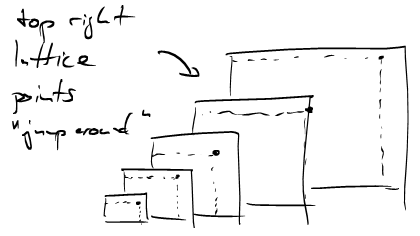This leads us to the following observation:

The sums $s'(a,b;c) := \sum_{k=0}^{c-1} \left\{\frac{ka}{c}\right\}\left\{\frac{kb}{c}\right\}$ measure nothing but the area of the small rectangle between $(\lfloor \frac{ka}{c} \rfloor, \lfloor \frac{kb}{c} \rfloor, k)$ and $(\frac{ka}{c},\frac{kb}{c},k)$.

Before we look at a few examples, a short remark on the fractional-part function:

Decomposing a fraction $\frac{a}{b}$ into its integral and fractional part $\frac{a}{b} = \left\lfloor \frac{a}{b} \right\rfloor + \left\{ \frac{a}{b} \right\}$ amounts to the same thing as diving $a$ by $b$ with remainder: $a = (a \text{ div } b)\cdot b +(a \text{ mod } b).$

This gives us $\left\lfloor\frac{a}{b} \right\rfloor = a \text{ div } b \;\;\;\text{ and }\;\;\; \left\{ \frac{a}{b} \right\} = \frac{a \text{ mod } b}{b}.$

Of course this is just notation. But to me, it serves as a reminder that we are doing just modular arithmetic here and not dealing with "real" real numbers. Also, we get $s'(a,b;c) = \frac{1}{c^2} \sum_{k=0}^{c-1} (ka \text{ mod }c)\cdot(kb\text{ mod } c)$ and can read off that $c^2\cdot s'(a,b;c)$ is integral - so it counts something!

Let us now visualize $c^2\cdot s'(a,b;c)$ by drawing the "rectangles" $(ka \text{ mod }c)\cdot(kb\text{ mod } c)$ for $k=0,\ldots,c-1$. We pick the example $7^2\cdot s'(5,1;7) = 5\cdot 1 + 3 \cdot 2 + 1 \cdot 3 + 6 \cdot 4 + 4 \cdot 5 + 2 \cdot 6.$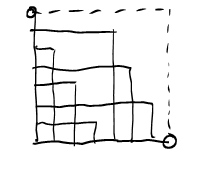How does the "corner" defining the rectangle move when we pass from one column $k$ to the next? As we are doing modular arithmetic here, we are operating on a torus here. We can see this by identifying the top and bottom, and left and right edges of this square, respectively. Moving from one corner to the next corresponds to translation by the vector $(5,1)$ on the torus. So the corners of these rectangles all lie on one line.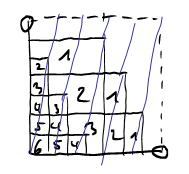In the above image, each region is labled with a number. This number gives the number of rectangles this region is contained in. If we now consider all sums $s'(a,1;7)$ for $a=1,\ldots,6$ we get all (linear) lines $\text{mod }7$.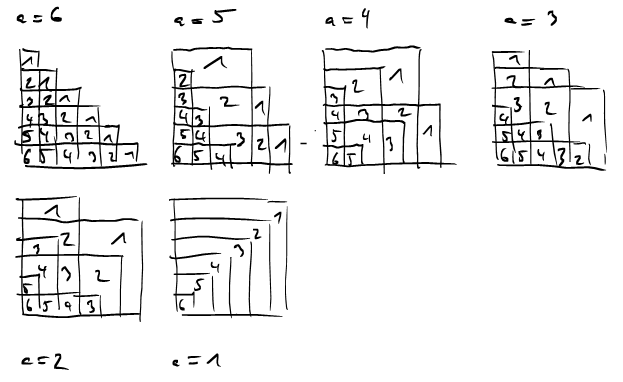Note that in the relatively prime case it suffices to consider sums of the form $s'(a,1;c)$ because $s'(a,b;c) = s'(ab^{-1},1;c)$ where $b^{-1}$ denotes the multiplicative inverse of $b$ modulo $c$. Can you see this in the picture?

Now, here are two questions for you:

Question. Does this visualization give an immediate geometric proof of Dedekind reciprocity? Why do $s'(a,1;b)$ and $s'(b,1;a)$ sum to something simple?

Question. We have proved a reciprocity law for the "pyramid sums" $L(\hat{P}_{a,b;c})$. Is this reciprocity law equivalent to some classic reciprocity law for Dedekind sums and their generalizations?

For inspiration in this regard, you may want to consult Chapter 8 of Computing the Continuous Discretely by Matthias Beck and Sinai Robins.

## Pyramids with Lattice Points on the Boundary

I promised to work out the reciprocity law for $L(\hat{P}_{a,b;c})$ when $a,b,c$ are not relatively prime. To this end we need to work out the number of lattice points in the triangle $P_{a,b;c} \cap P_{a,c;b} =\text{conv}( \;(0,0,0), \; (0,b,c) ,\; (a,b,c) \; ).$

We want to find a lattice transformation that maps this triangle into one of our coordinate hyperplanes, so that we can apply last lecture’s formula for counting lattice points. Let’s look at this triangle from the side, along the $x_1$-axis. Then it looks just like a line from $(0,0,0)$ to $(0,b,c)$. This line contains $\text{gcd}(b,c)+1$ lattice points. The "first" lattice point after the origin has coordiantes $(0,\frac{b}{\text{gcd}(b,c)},\frac{c}{\text{gcd}(b,c)})$. We would now like to map this line onto the (in this picture) horizontal axis, such that $(0,b,c)$ is mapped to $(0,0,\text{gcd}(a,b))$, so that the $\text{gcd}(b,c)+1$ lattice points on the line are mapped to $\text{gcd}(b,c)+1$ adjacent lattice points on the $x_3$-axis. We do not want to affect the $x_1$-coordinate at all, so we, similarly, want to map $(a,b,c)$ to $(a,0,\text{gcd}(b,c))$.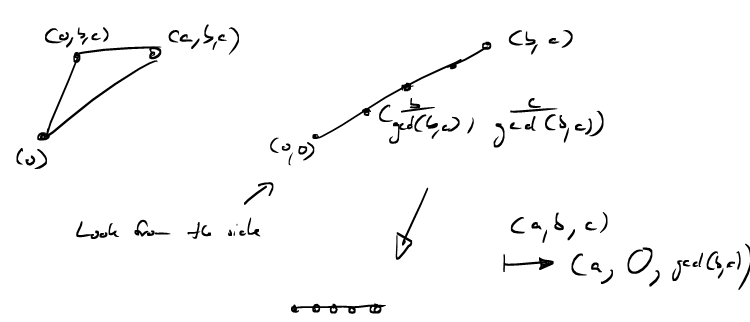Let’s work out the matrix for this lattice transformation. We want $\begin{pmatrix} ? & ? & ? \\ ? & ? & ? \\ ? & ? & ? \end{pmatrix} \cdot \begin{pmatrix} a \\ b \\ c \end{pmatrix} = \begin{pmatrix} a \\ 0 \\ \text{gcd}(b,c) \end{pmatrix}.$

As the first coordinate is supposed to remain invariant, we put $\begin{pmatrix} 1 & 0 & 0 \\ 0 & ? & ? \\ 0 & ? & ? \end{pmatrix} \cdot \begin{pmatrix} a \\ b \\ c \end{pmatrix} = \begin{pmatrix} a \\ 0 \\ \text{gcd}(b,c) \end{pmatrix}.$

How can we combine $b$ and $c$ to get $\text{gcd}(b,c)$? This is precisely what the extended Euclidean algorithm does for us. It computes integers $x$ and $y$ such that $xb+yc=\text{gcd}(b,c)$ which gives us $\begin{pmatrix} 1 & 0 & 0 \\ 0 & ? & ? \\ 0 & x & y \end{pmatrix} \cdot \begin{pmatrix} a \\ b \\ c \end{pmatrix} = \begin{pmatrix} a \\ 0 \\ \text{gcd}(b,c) \end{pmatrix}.$

Now we want to fill in the second row such that $b$ and $c$ cancel out. A good first idea is $\begin{pmatrix} 1 & 0 & 0 \\ 0 & -c & b \\ 0 & x & y \end{pmatrix} \cdot \begin{pmatrix} a \\ b \\ c \end{pmatrix} = \begin{pmatrix} a \\ 0 \\ \text{gcd}(b,c) \end{pmatrix}.$

But this matrix does not have all the properties we would like. We also want it to be a lattice transform, so in particular, its determinant has to be $\pm 1$. Currently, however, the determinant is $-cy-bx=-\text{gcd}(b,c).$ So what we have to do is divide those two entries by $\text{gcd}(b,c)$ to give us our final matrix:

$\begin{pmatrix} 1 & 0 & 0 \\ 0 & -\frac{c}{\text{gcd}(b,c)} & \frac{b}{\text{gcd}(b,c)} \\ 0 & x & y \end{pmatrix} \cdot \begin{pmatrix} a \\ b \\ c \end{pmatrix} = \begin{pmatrix} a \\ 0 \\ \text{gcd}(b,c) \end{pmatrix}.$

This is a lattice transform and it maps

$\begin{pmatrix} 1 & 0 & 0 \\ 0 & -\frac{c}{\text{gcd}(b,c)} & \frac{b}{\text{gcd}(b,c)} \\ 0 & x & y \end{pmatrix} \cdot \begin{pmatrix} 0 \\ \frac{b}{\text{gcd}(b,c)} \\ \frac{c}{\text{gcd}(b,c)} \end{pmatrix} = \begin{pmatrix} 0 \\ 0 \\ 1 \end{pmatrix}$

and

$\begin{pmatrix} 1 & 0 & 0 \\ 0 & -\frac{c}{\text{gcd}(b,c)} & \frac{b}{\text{gcd}(b,c)} \\ 0 & x & y \end{pmatrix} \cdot \begin{pmatrix} 0 \\ -y \\ x \end{pmatrix} = \begin{pmatrix} 0 \\ 1 \\ 0 \end{pmatrix}.$

So the lattice point $(0,-y,x)$ corresponds to the standard unit vector $(0,1,0)$ in this bijection. Can we interpret this somehow?

Let’s look at an example. Let $b=10$ and $c=14$ such that $\text{gcd}(b,c)=2$. Then $3 \cdot 10 - 2 \cdot 14 = 2$ so $x= 3$ and $y=-2$ and the mysterious lattice point is $(0,2,3)$.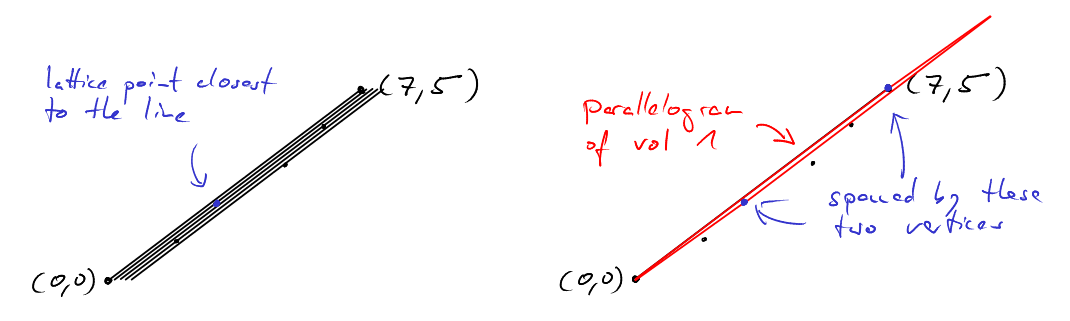In this picture there are two things to notice:

1. Consider all translates of the line through $(0,0)$ and $(10,14)$ that go through some lattice point. $(2,3)$ lies on the translate closest to the original.
2. The vectors $(2,3)$ and $(5,7)$ span a parallelogram of volume 1.

These are no accidents. $(2,3)$ and $(5,7)$ form a lattice basis. And the task of finding a lattice transformation to the standard basis is equivalent to finding a lattice basis. Let us make these notions precise.

## Lattice Bases

Recall that a lattice transformation $f:\mathbb{R}^d\rightarrow\mathbb{R}^d$ is an affine isomorphism that, when restricted to the integer lattice $\mathbb{Z}^d$, induces a bijection (i.e., a 1-to-1 and onto map) on the integer lattice $f|_{\mathbb{Z}^d}: {\mathbb{Z}^d} \rightarrow {\mathbb{Z}^d}$. Now, a lattice basis is a set $a_1,\ldots,a_d\in\mathbb{Z}^d$ of linearly independent integer vectors such that for every $z\in\mathbb{Z}^d$ there exist integers $\lambda_1,\ldots,\lambda_d\in\mathbb{Z}$ such that $\sum_{i=1}^d \lambda_i a_i = z.$

These notions are closely related. For linearly independent integer vectors $a_1,\ldots,a_d\in\mathbb{Z}^d$ the following are equivalent:

• $a_1,\ldots,a_d$ form a lattice basis.
• There exists a lattice transformation $f$ such that $f(a_i)=e_i$ for $i=1,\ldots,d$ where $e_i$ are the standard unit vectors.
• The fundamental parallelepiped $\Pi_{a_1,\ldots,a_d}$ contains exactly one lattice point $z\in\mathbb{Z}^d$
Here, the fundamental parallelepiped $\Pi_{a_1,\ldots,a_d}$ spanned by $a_1,\ldots,a_d$ is the set
$\Pi_{a_1,\ldots,a_d}= \{ x\in\mathbb{R}^d \; | \; x = \sum_{k=1}^d \lambda_i a_i \text{ for } 0 \leq \lambda_i < 1 \}.$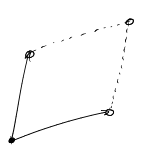The crucial ingredient to the proof of the above equivalences is the following theorem.

Theorem. Let $a_1,\ldots,a_d\in\mathbb{Z}^d$ be linearly independent integer vectors. Let $A=(a_1 \; \ldots \;a_d)$ be the matrix with the $a_i$ as columns. Then $L(\Pi_{a_1,\ldots,a_d}) = \text{Volume}(\Pi_{a_1,\ldots,a_d}) = |\det(A)|.$

Now, as we will see, it is always the case that the lattice point count approximates the volume in a certain sense. But for fundamental parallelepipeds we have equality here! The reason is the following.

Sketch of Proof. First of all we make the approximation argument a bit more precise, by arguing that

$\lim_{k\rightarrow\infty} \frac{1}{2^{kd}}|\frac{1}{2^k}\mathbb{Z}^d \cap \Pi_{a_1,\ldots,a_d}| = Volume(\Pi_{a_1,\ldots,a_d}).$

Instead of counting lattice points in some set $X$, we can also measure the volume of a set of unit cubes, that each have one of these lattice points at their center. Intuitively, this is a rough approximation of the volume of the set.

Next, we refine this approximation in the following way. We refine the lattice $\mathbb{Z}^d$ by scaling it by $\frac{1}{2}$. Now we play the same game with the cubes, measuring the volume of a set of cubes, each with one of the lattice points in $X$ at its center. To make sure that the cubes don’t overlap we have to scale their sidelengths by $\frac{1}{2}$ as well, which scales their volume by $\frac{1}{2^d}$.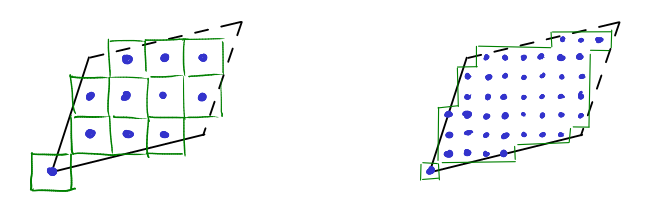If we continue in this fashion, we our cube set in the $k$th step will have volume $\frac{1}{2^{kd}}|\frac{1}{2^k}\mathbb{Z}^d \cap \Pi_{a_1,\ldots,a_d}|$. And we can see that the volume of this cube set approximates the volume of the parallelepiped, in the same way that the number of pixels, multiplied by the area of a pixel, will approximate the area of a parallelogram drawn on a (very high resolution) computer screen.

So far, this works for all polytopes, not just for the parallelepiped. But now comes the curcial property of the fundamental parallelepiped.

$L(k\cdot\Pi_{a_1,\ldots,a_d}) = k^d \cdot L(\Pi_{a_1,\ldots,a_d})$

The reason is simply that we can tile the $k$-th dilate of $\Pi_{a_1,\ldots,a_d}$ by $k^d$ integral translates of $\Pi_{a_1,\ldots,a_d}$. Because these are integral translates, they all contain the same number of lattice points as the original fundamental parallelepiped.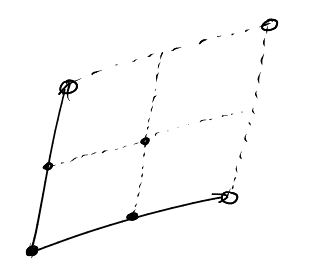Now all we have to observe is that refining the lattice is the same as dilating our parallelepiped. Then, our proof is simply this:

$L(\Pi_{a_1,\ldots,a_d}) =\lim_{k\rightarrow\infty} \frac{1}{2^{kd}} L(2^k\cdot \Pi_{a_1,\ldots,a_d}) = \lim_{k\rightarrow\infty} \frac{1}{2^{kd}} |\frac{1}{2^k}\mathbb{Z}^d \cap \Pi_{a_1,\ldots,a_d}| = \text{Volume}(\Pi_{a_1,\ldots,a_d}).$

## Conclusion

After this detour, let us finally put our reciprocity theorem in the non-relatively prime case together.

Using our lattice transformation, we have seen that $L(\text{conv}( \;(0,0,0), \; (0,b,c) ,\; (a,b,c) \; )) = L(\text{conv}( \;(0,0,0), \; (0,0,\text{gcd}(b,c)) ,\; (a,0,\text{gcd}(b,c)) \; ))$

Using our formula from the last lecture, we conclude that

$L(\text{conv}( \;(0,0,0), \; (0,0,\text{gcd}(b,c)) ,\; (a,0,\text{gcd}(b,c)) \; )) = \frac{(a+1)\text{gcd}(b,c) + \text{gcd}(a,b,c) + 1}{2}$

We now have to adjust for the fact that the pyramids $\hat{P}_{a,b;c}$ are half open. In particular, the two "rectangular" sides have been removed (when intersecting half open pyramids). This gives us

$L(\hat{P}_{a,b;c}\cap \hat{P}_{a,c;b}) = \frac{(a+1)\text{gcd}(b,c) + \text{gcd}(a,b,c) + 1}{2} - a - \text{gcd}(b,c) - 1$

which simplifies to

$L(\hat{P}_{a,b;c}\cap \hat{P}_{a,c;b}) = \frac{1}{2}\left((\text{gcd}(b,c) -2) a - \text{gcd}(b,c) + \text{gcd}(a,b,c) -1\right).$

These observations also allow us to compute the number of lattice points on the diagonal

$L(\hat{P}_{a,b;c}\cap \hat{P}_{a,c;b} \cap \hat{P}_{b,c;a}) = \text{gcd}(a,b,c) -1.$

Now we can out all of these formulas together by applying inclusion-exclusion.

$\begin{eqnarray} (a-1)(b-1)(c-1) & = & L(\hat{P}_{a,b;c}) + L(\hat{P}_{a,c;b}) + L(\hat{P}_{b,c;a}) \\ && - L(\hat{P}_{a,b;c}\cap \hat{P}_{a,c;b}) - L(\hat{P}_{a,b;c}\cap \hat{P}_{b,c;a}) - L(\hat{P}_{a,c;b}\cap \hat{P}_{b,c;a}) \\ && + L(\hat{P}_{a,b;c}\cap \hat{P}_{a,c;b} \cap \hat{P}_{b,c;a}) \end{eqnarray}$

This yields

And all of this, just because we fit three pyramids together to form a quader!

While the first two lectures focused on two examples of how visualizing numbers can yield actual formulas, we are going to start with some theory in the next lecture and talk about polytopes. So you do not have to worry: the next lecture is not about $\sum_k k^3$! Here is another question, though:

Can you work out the classic formula for $\sum_k k^d$ by putting $d$ pyramids over a $d-1$ cube together?